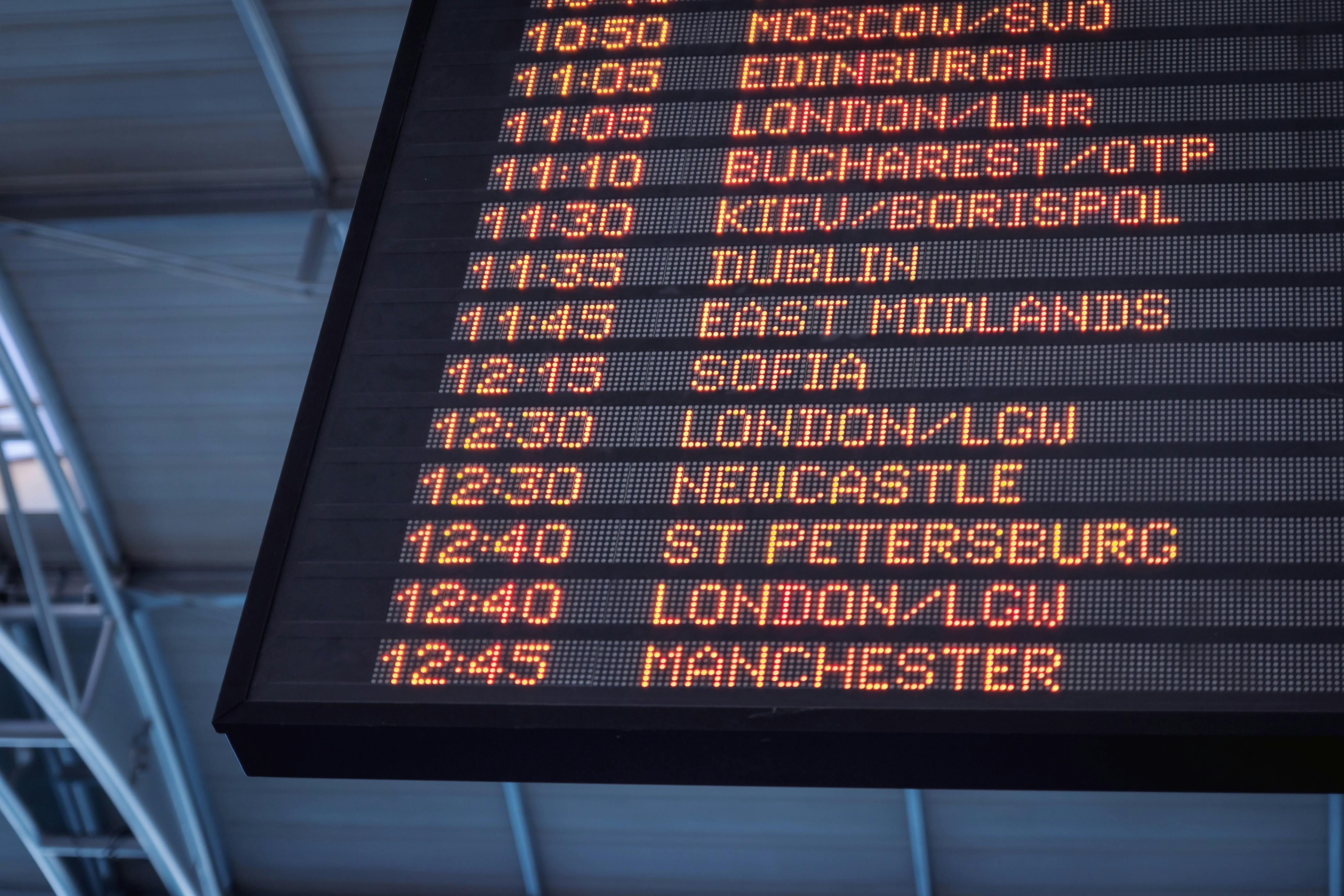Airline Status Planner
Share
ExploreWill you qualify?
false
-
I'm sorry. Doesn't look
like you will make
status
EQM
check?
false
EQS
check?
false
EQD
check?
false
Credit Card spend check?

Enter your planned flights for the year below: You can calculate the Distance with generated links below
Flights
0
Search
From
To
Airline
Fare Class
Calculate Distance
Distance
Dollars
1
SFO
DTW
DL
K
🛩 Calculate Distance
2,079
\$108.00
2
DTW
AMS
DL
K
🛩 Calculate Distance
3,941
\$208.00
3
AMS
CPT
DL
X
🛩 Calculate Distance
5,998
\$316.00
4
CPT
AMS
DL
X
🛩 Calculate Distance
5,998
\$316.00
5
AMS
SEA
DL
K
🛩 Calculate Distance
4,887
\$258.00
6
SEA
SFO
DL
X
🛩 Calculate Distance
679
\$36.00
7
JFK
BOS
DL
E
🛩 Calculate Distance
187
\$50.00
8
BOS
SFO
DL
E
🛩 Calculate Distance
2,705
\$200.00
There are no rows in this table
26,474
Sum
\$1,492.00
Sum